## A potter made a mud vessel, where the shape of the pot is based on f(x)=|x-3|+|x-2|, where f(x) represents the height of the pot.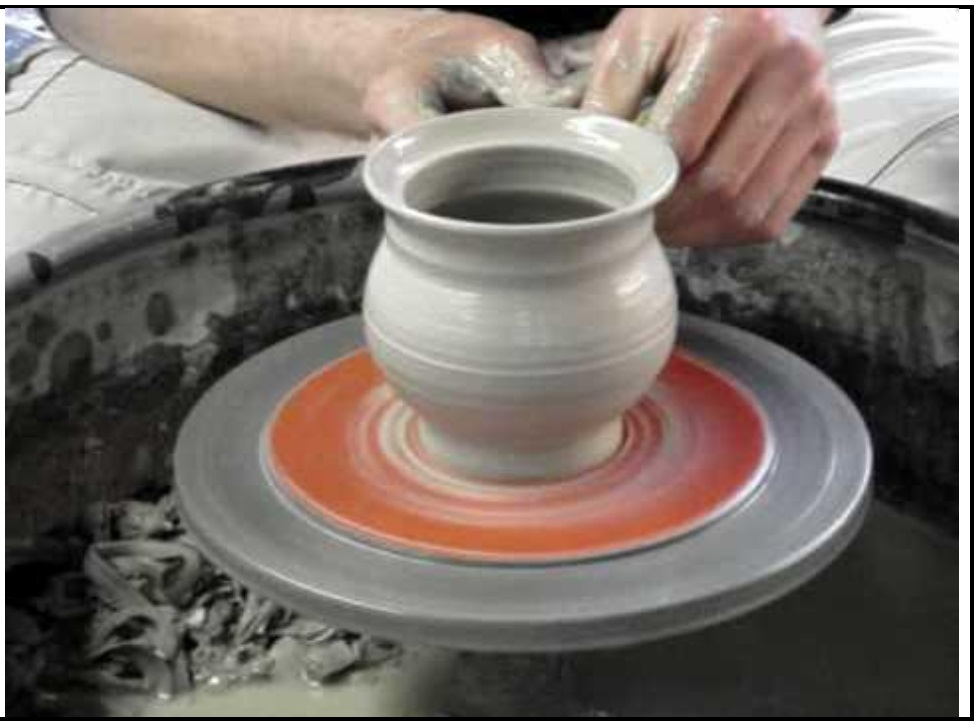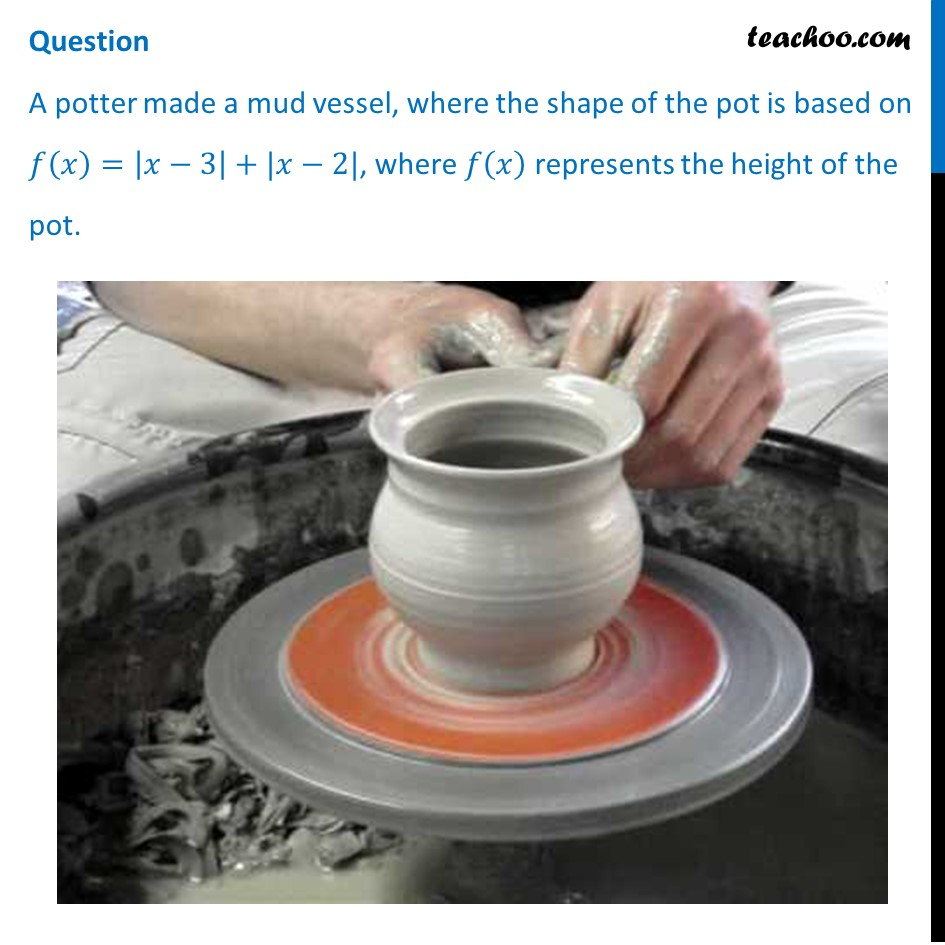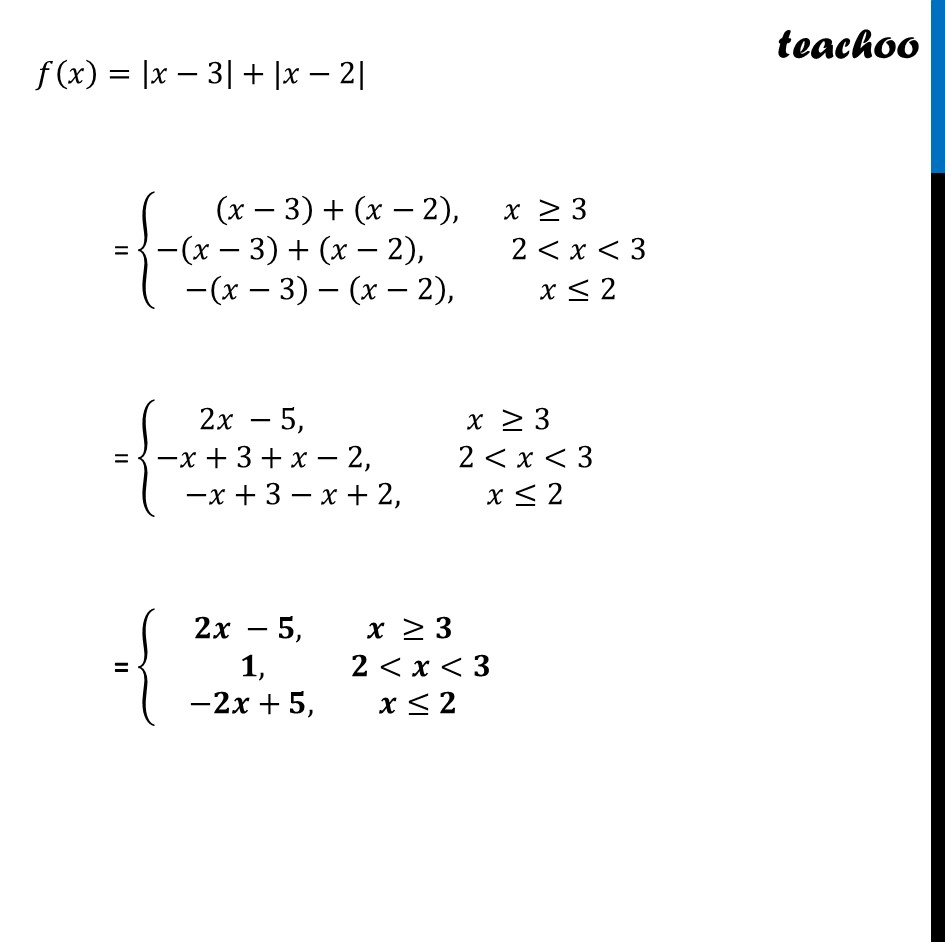## (d) 5 – 2x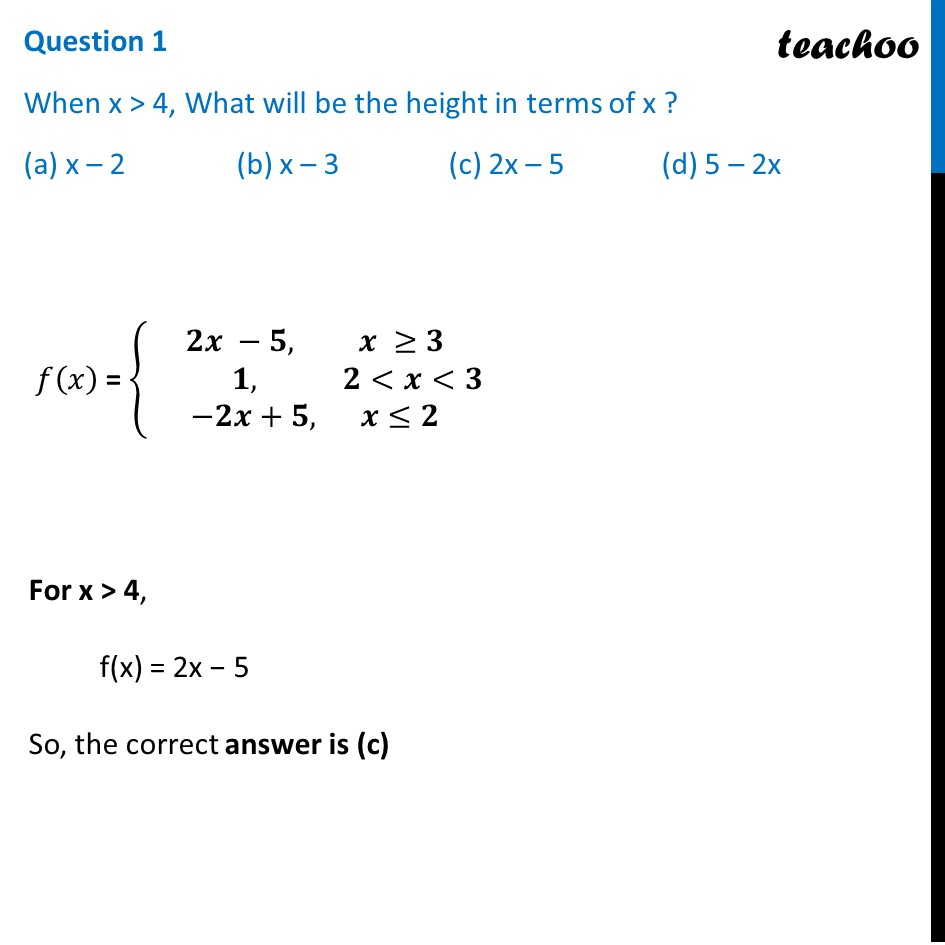## (b) No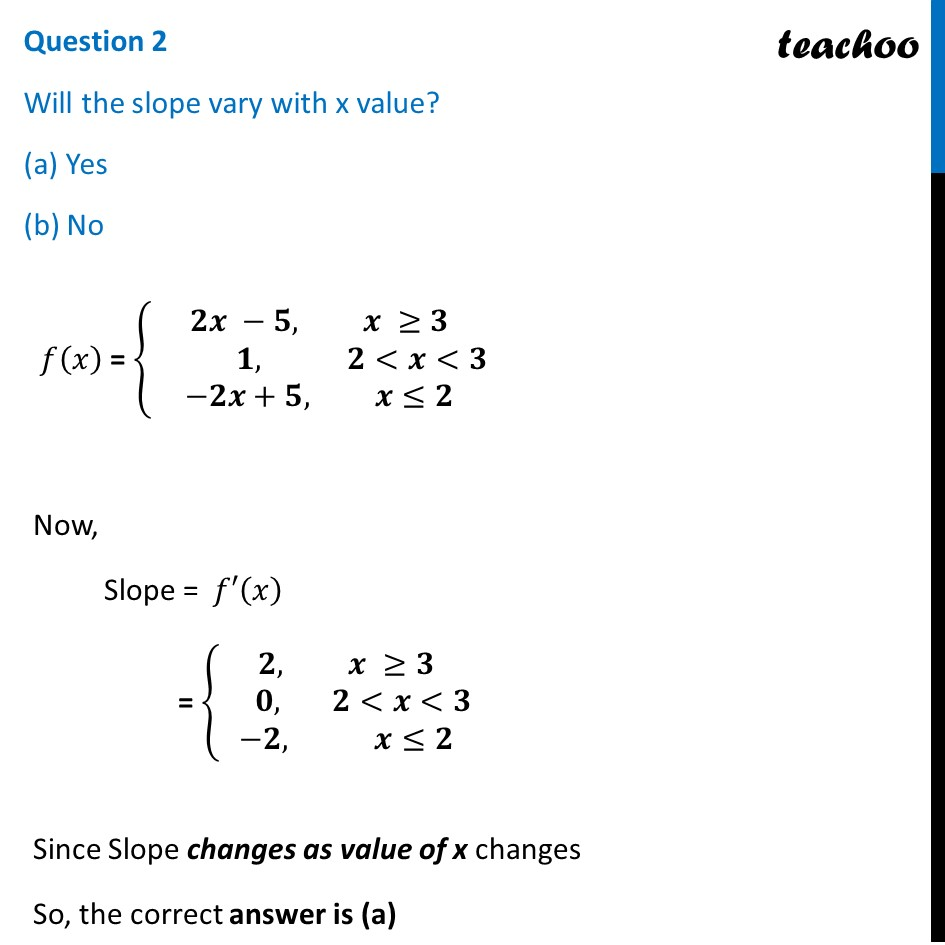## (d) 1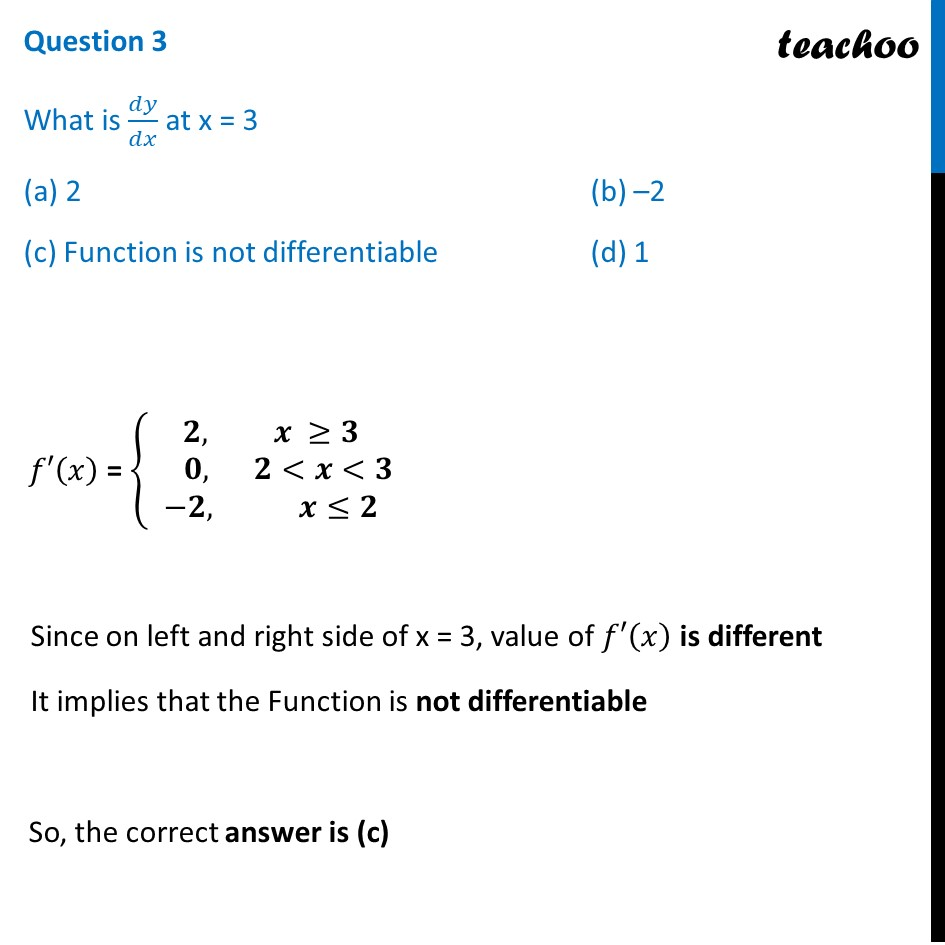## (d) 5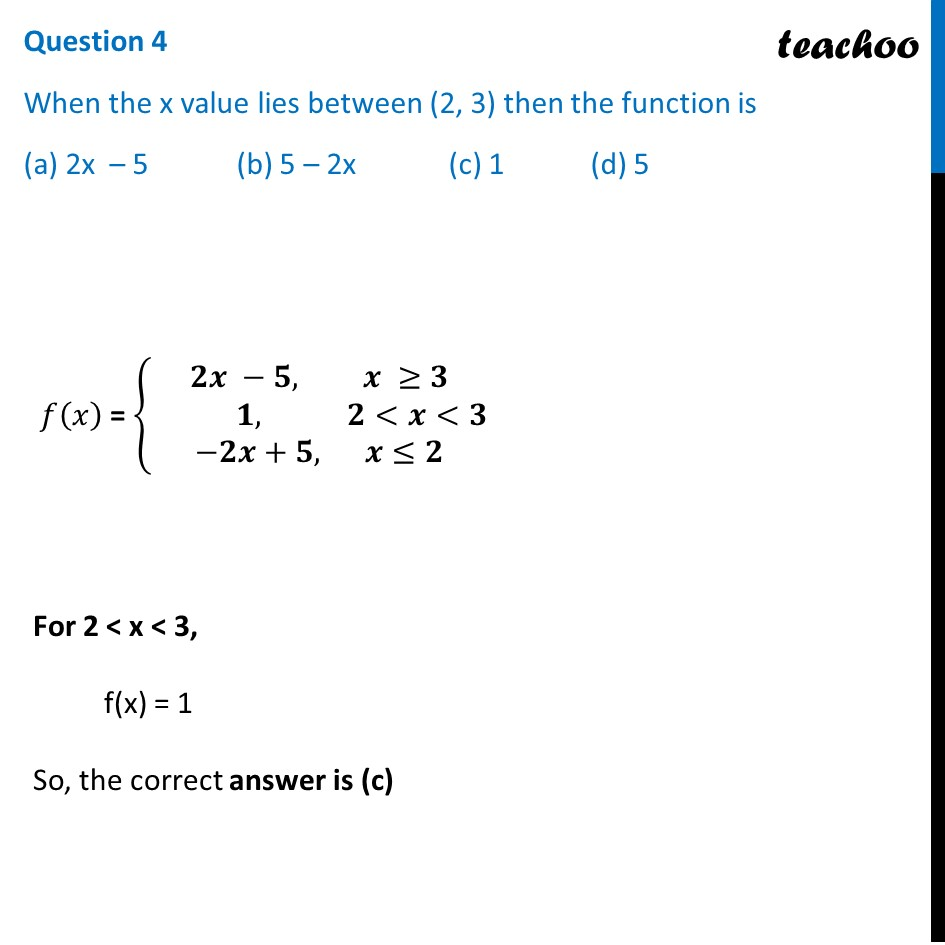## (d) No, because it is not continuous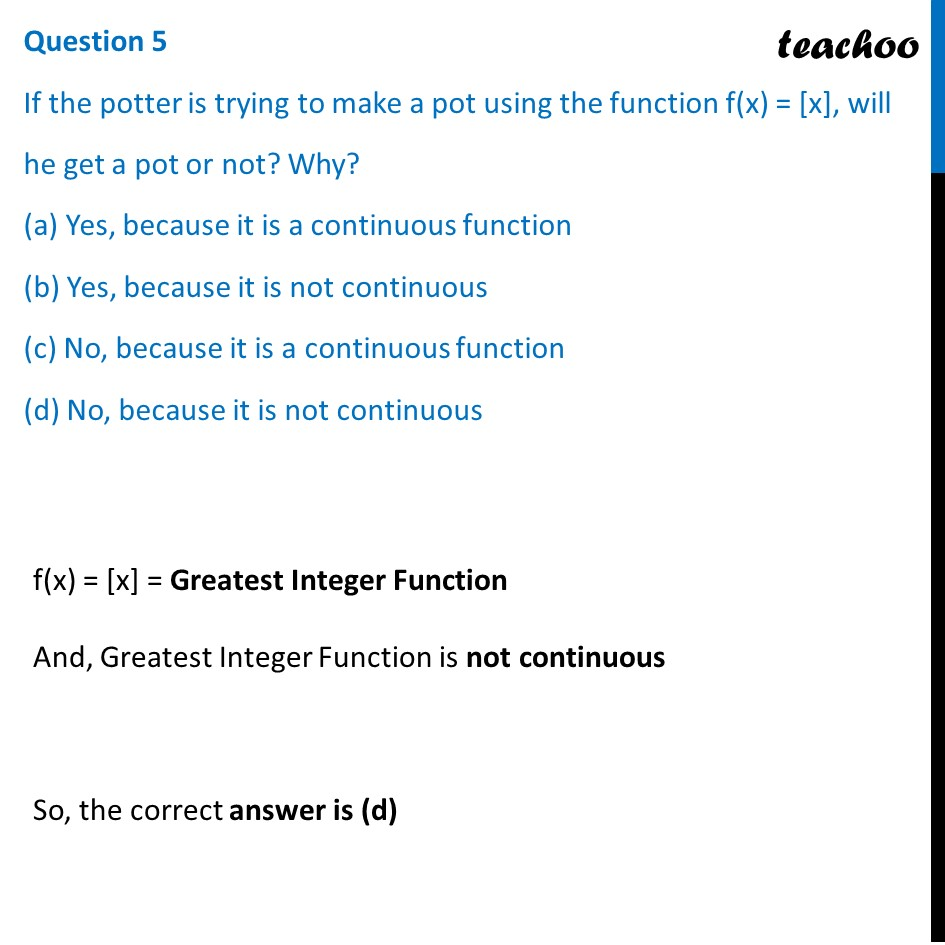1. Chapter 6 Class 12 Application of Derivatives (Term 1)
2. Serial order wise
3. Case Based Questions (MCQ)

Transcript

Question A potter made a mud vessel, where the shape of the pot is based on 𝑓(𝑥)=|𝑥−3|+|𝑥−2|, where 𝑓(𝑥) represents the height of the pot. 𝑓(𝑥)=|𝑥−3|+|𝑥−2| = {█((𝑥−3)+(𝑥−2), 𝑥 ≥3@−(𝑥−3)+(𝑥−2), 2<𝑥<3@−(𝑥−3)−(𝑥−2), 𝑥≤2)┤ = {█(2𝑥 −5, 𝑥 ≥3@−𝑥+3+𝑥−2, 2<𝑥<3@−𝑥+3−𝑥+2, 𝑥≤2)┤ = {█(𝟐𝒙 −𝟓, 𝒙 ≥𝟑@ 𝟏, 𝟐<𝒙<𝟑@−𝟐𝒙+𝟓, 𝒙≤𝟐)┤ Question 1 When x > 4, What will be the height in terms of x ? (a) x – 2 (b) x – 3 (c) 2x – 5 (d) 5 – 2x 𝑓(𝑥) = {█(𝟐𝒙 −𝟓, 𝒙 ≥𝟑@ 𝟏, 𝟐<𝒙<𝟑@−𝟐𝒙+𝟓, 𝒙≤𝟐)┤ For x > 4, f(x) = 2x − 5 So, the correct answer is (c) Question 2 Will the slope vary with x value? (a) Yes (b) No 𝑓(𝑥) = {█(𝟐𝒙 −𝟓, 𝒙 ≥𝟑@ 𝟏, 𝟐<𝒙<𝟑@−𝟐𝒙+𝟓, 𝒙≤𝟐)┤ Now, Slope = 𝑓′(𝑥) = {█(𝟐, 𝒙 ≥𝟑@ 𝟎, 𝟐<𝒙<𝟑@−𝟐, 𝒙≤𝟐)┤ Since Slope changes as value of x changes So, the correct answer is (a) Question 3 What is 𝑑𝑦/𝑑𝑥 at x = 3 (a) 2 (b) –2 (c) Function is not differentiable (d) 1 𝑓′(𝑥) = {█(𝟐, 𝒙 ≥𝟑@ 𝟎, 𝟐<𝒙<𝟑@−𝟐, 𝒙≤𝟐)┤ Since on left and right side of x = 3, value of 𝑓′(𝑥) is different It implies that the Function is not differentiable So, the correct answer is (c) Question 4 When the x value lies between (2, 3) then the function is (a) 2x – 5 (b) 5 – 2x (c) 1 (d) 5 𝑓(𝑥) = {█(𝟐𝒙 −𝟓, 𝒙 ≥𝟑@ 𝟏, 𝟐<𝒙<𝟑@−𝟐𝒙+𝟓, 𝒙≤𝟐)┤ For 2 < x < 3, f(x) = 1 So, the correct answer is (c) Question 5 If the potter is trying to make a pot using the function f(x) = [x], will he get a pot or not? Why? (a) Yes, because it is a continuous function (b) Yes, because it is not continuous (c) No, because it is a continuous function (d) No, because it is not continuous f(x) = [x] = Greatest Integer Function And, Greatest Integer Function is not continuous So, the correct answer is (d)

Case Based Questions (MCQ)